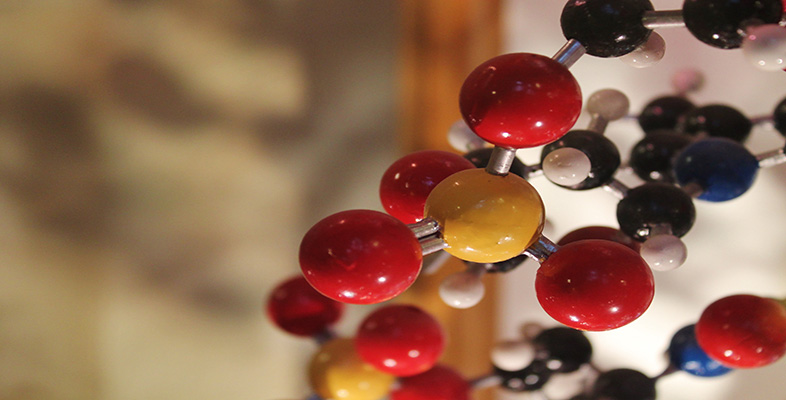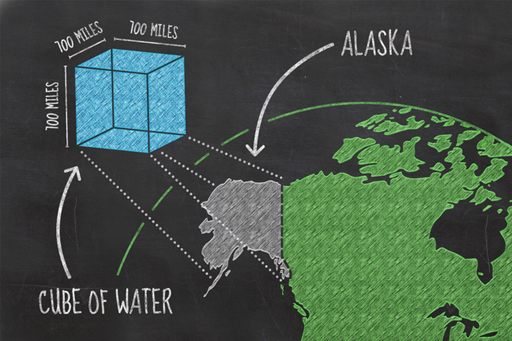Basic science: understanding numbers

Start this free course now. Just create an account and sign in. Enrol and complete the course for a free statement of participation or digital badge if available.

Free course

# 1.8 Big things, big numbersFigure _unit1.8.1 Figure 6 If you gathered all the water from the world and shaped it into a cube, how big would it be?

Scientific notation helps express large numbers but sometimes it’s difficult to imagine such large things. The best way to grasp the size of something you can’t see is to compare it with something you already know – can you remember how many swimming pools full of bottled water we drink per year?

## Big things

Most of the Earth’s water is found in the oceans, about 97% of it; 3% of the remaining water is frozen in glaciers and icecaps, some is in the ground, and in lakes, swamps and rivers. A satellite image of the Earth shows that some two-thirds of the surface is covered in water. But that area (361,132,000 km2 or 3.61 × 108 km2, incidentally) can mislead you as to how much water there really is. If you could gather all of the water on Earth, from the oceans, the icecaps, the lakes and rivers, and create one huge cube, how big do you think that cube would be?

It turns out that it would be a cube about 1135 km (or 700 miles) along each side. To give that some context, it could sit on the footprint of Alaska, which covers around 0.3% of the surface of the Earth. So, now you have a mental image of the volume of the Earth’s water, and also the means to work out the depth of the oceans. Not feeling like it? Don’t worry, it’s coming up next week anyway, so you can sit back and wait.

## Big numbers

Large numbers are required to discuss large things. The total amount of water on the Earth is a large number, it’s estimated to be 1.46 × 1021 litres or written out in full, that is 1,460,000,000,000,000,000,000 litres.

Well, we have been given a number in units of litres, but litres are quite small in comparison to the world’s oceans, so it would be useful to convert them to a unit that is more useful, such as cubic kilometres. A cubic kilometre is a cube with dimensions of 1 km × 1 km × 1 km.

Given that a litre of water occupies the space of a 10 cm × 10 cm × 10 cm cube (neat how SI units make the maths easier, isn’t it?), you can first work out how many litres are in a cubic metre, that is, a volume with dimensions of 1 m × 1 m × 1 m (or, to put it another way, 100 cm × 100 cm × 100 cm).

If you imagine laying litre-sized (10 cm × 10 cm × 10 cm) blocks on the floor in a row, lining ten one litre cubes in a row, then another line of ten, then another, and so on, you would cover the bottom layer of a one metre cube with ten rows of ten, a total of a hundred of them.

You would then need to build another layer of a hundred cubes on top of that, then again for a total of ten times to completely build up a cube with dimensions of 1 m × 1 m × 1 m. This would require 1,000 × 1 litre cubes. To put this in scientific notation, 1 m3 = 1000 or 1 × 103 litres.

The original 1,460,000,000,000,000,000,000 (or 1.46 × 1021) litres on the Earth can also be written as 1,460,000,000,000,000,000 (or 1.46 × 1018) cubic metres.

You can repeat this to work out how many cubic metres it will take to fill a cube that is 1 kilometre on each side. This time, you need a row of 1,000 at the bottom of the larger cube, and the bottom layer will be 1,000 rows of 1,000 cubic metres. It will take a further thousand similar layers to fill the larger cube. You can see that it takes 1,000 × 1,000 × 1,000 cubic metres to fill a cubic kilometre. 1,000 × 1,000 × 1,000 is 1,000,000,000, or 109.

You can now convert our 1,460,000,000,000,000,000 (or 1.46 × 1018) cubic metres to 1,460,000,000 (or 1.46 × 109) cubic kilometres. As before we can just subtract the superscript number or power, 18 - 9 = 9.

It turns out that a cube with dimensions of 1135 km × 1135 km × 1135 km comes pretty close to this volume, which brings us back to Alaska, and in truth this only covers the mainland of Alaska, which includes several of the Aleutian Isles and a long thin stretch of coastline on the western margin of Canada. Like all analogies it only goes so far, but you get the idea.